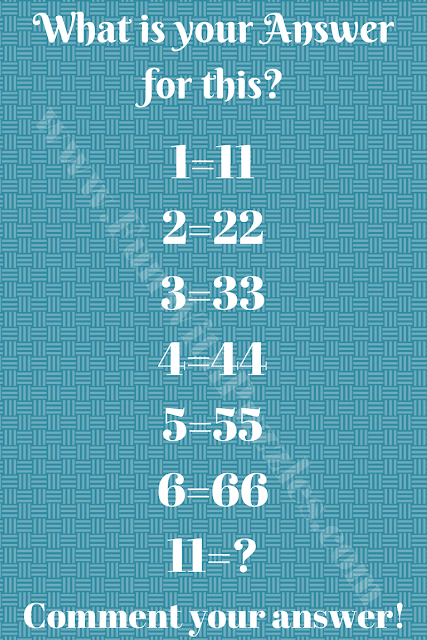This is a very interesting trick question. In this puzzle image, there are some number equations. You need to decode the hidden logic in these logical number equations. Once you are able to crack the hidden logic, find the value of the missing number that replaces the question mark in the last equation. Can You Answer this Tricky Puzzle Question?Can You Answer this Tricky Question?

tanmoy said...

121

Unknown said...

121
Because
1×11=11
2×11=22
....
...
...
6×11=66

tryhard05 said...

1111

Unknown said...

Guru said...

10

Unknown said...

1

Unknown said...

121

Unknown said...

121 because that's what number is multiplied
By 11 and it's answer so 11×11= 121